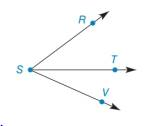Chapter 1.4, Problem 20E### Elementary Geometry for College St...

6th Edition
Daniel C. Alexander + 1 other
ISBN: 9781285195698

#### Solutions

Chapter
Section### Elementary Geometry for College St...

6th Edition
Daniel C. Alexander + 1 other
ISBN: 9781285195698
Textbook Problem
3 views

#Exercises 16 − 24 Given: m ∠ R S T = 5 ( x + 1 ) − 3 m ∠ T S V = 4 ( x − 2 ) + 3 m ∠ R S V = 4 ( 2 x + 3 ) − 7 Find: x     and m ∠ R S V

To determine

To find:

The value of x and the measure of the angle RSV.

Explanation

Given:

mRST=5(x+1)3mTSV=4(x2)+3mRSV=4(2x+3)7

The given figure is,

Definition:

If a point say M lies in the interior of PQR, then mPQM+mRQM=mPQR.

Calculation:

Consider the figure,

T is the interior point of the angle RSV.

mRST=5(x+1)3mTSV=4(x2)+3mRSV=4(2x+3)7

Use above mentioned angle addition postulate to solve for the value of x.

### Still sussing out bartleby?

Check out a sample textbook solution.

See a sample solution

#### The Solution to Your Study Problems

Bartleby provides explanations to thousands of textbook problems written by our experts, many with advanced degrees!

Get Started